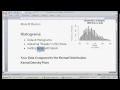### Simple Histograms with R SoftwareCreating a Histogram with R Software14:09  http://youtu.be/wGW9M93YswYIn this intro to R statistics video, we discuss the r script that makes histograms in R statistical software (R's hist function),  creating a kernal density plot, and (briefly) comparing two kernal densities.

I called my data data1

By typing str(data1),  you can see that the R object "data1", has three variables; section, class & final. (This happens to be data of student section attendance, lecture attendance and final exam scores for a class at Michigan State University.)

Simple Histogram
Let's create a histogram for their final exam grades:
hist(data1\$final)
This says, "draw a histogram by going into 'data1' and looking at the column 'final'". Note that you need the dollar-sign to link your R object 'data1' to the variable 'final'.

We get the following histogram:

Notice that there are only 9 bins to form the histogram above.
Since the maximum score was 166 points. let's set 166 bins so we have a vertical line for each possible exam score.

hist(data1\$final,166)

Well, it doesn't look great (I think my screen resolution was just really bad as I took the screen shot), but has a bit more information in it.

Adding Labels to a Histogram in R
Now, let's add some useful labels:
hist(data1\$final,100, main="Final Exam Test Scores", xlab="Exam Scores", ylab="Frequency")